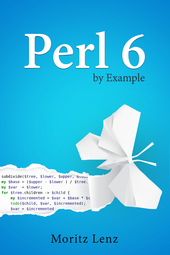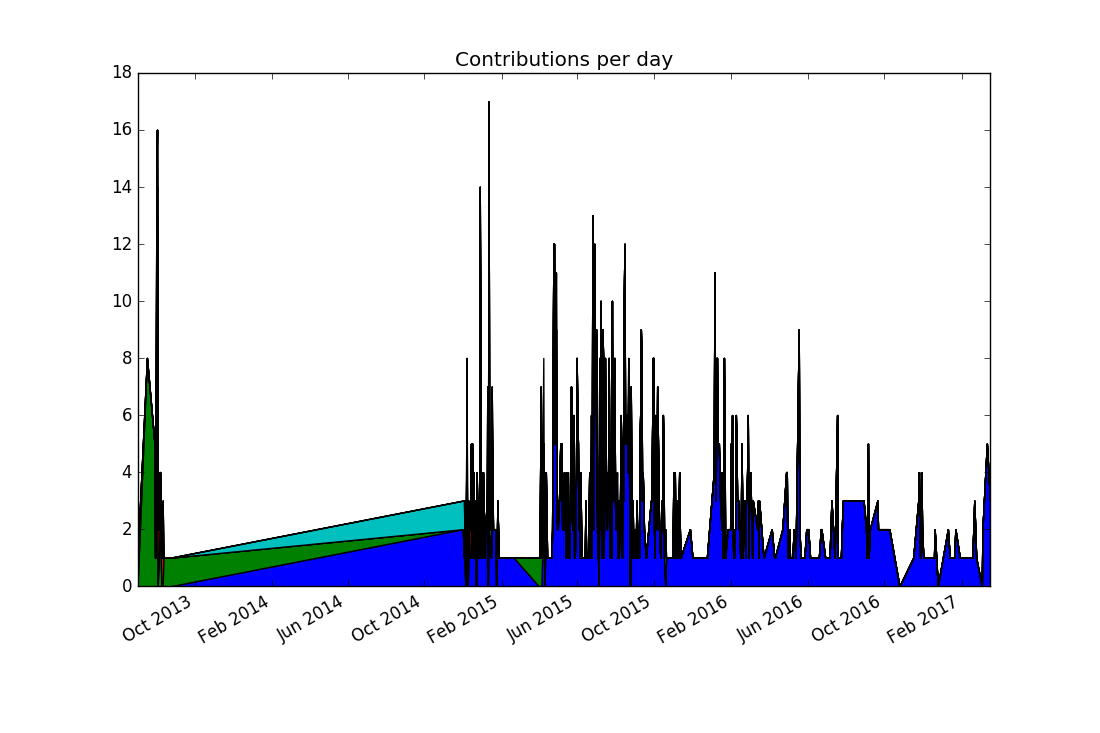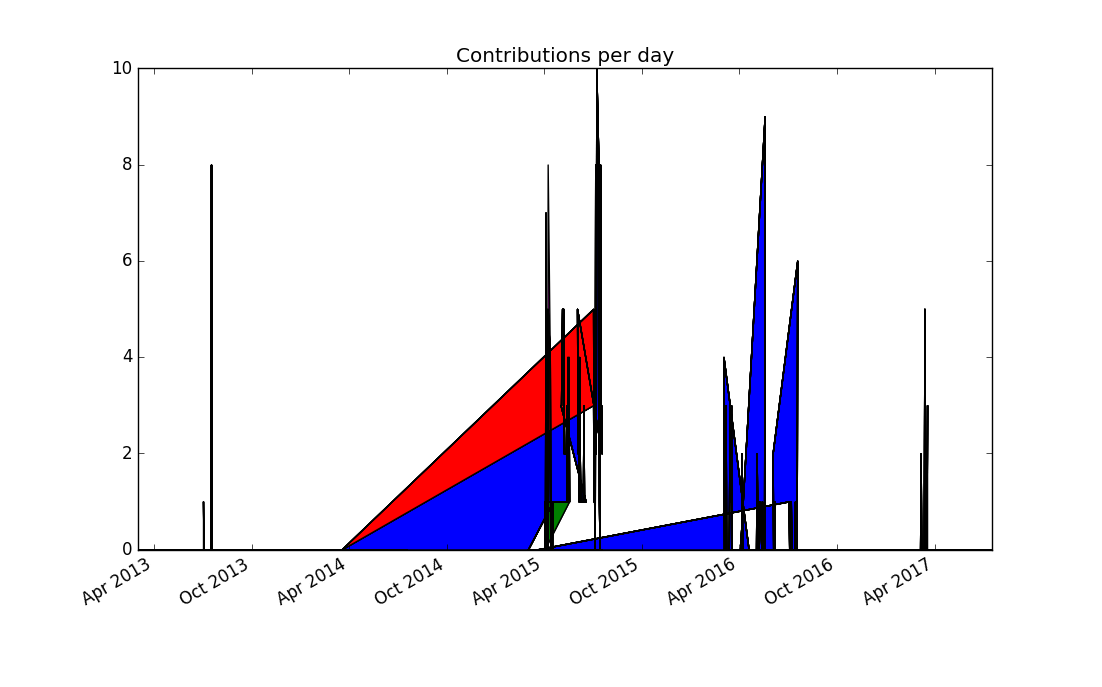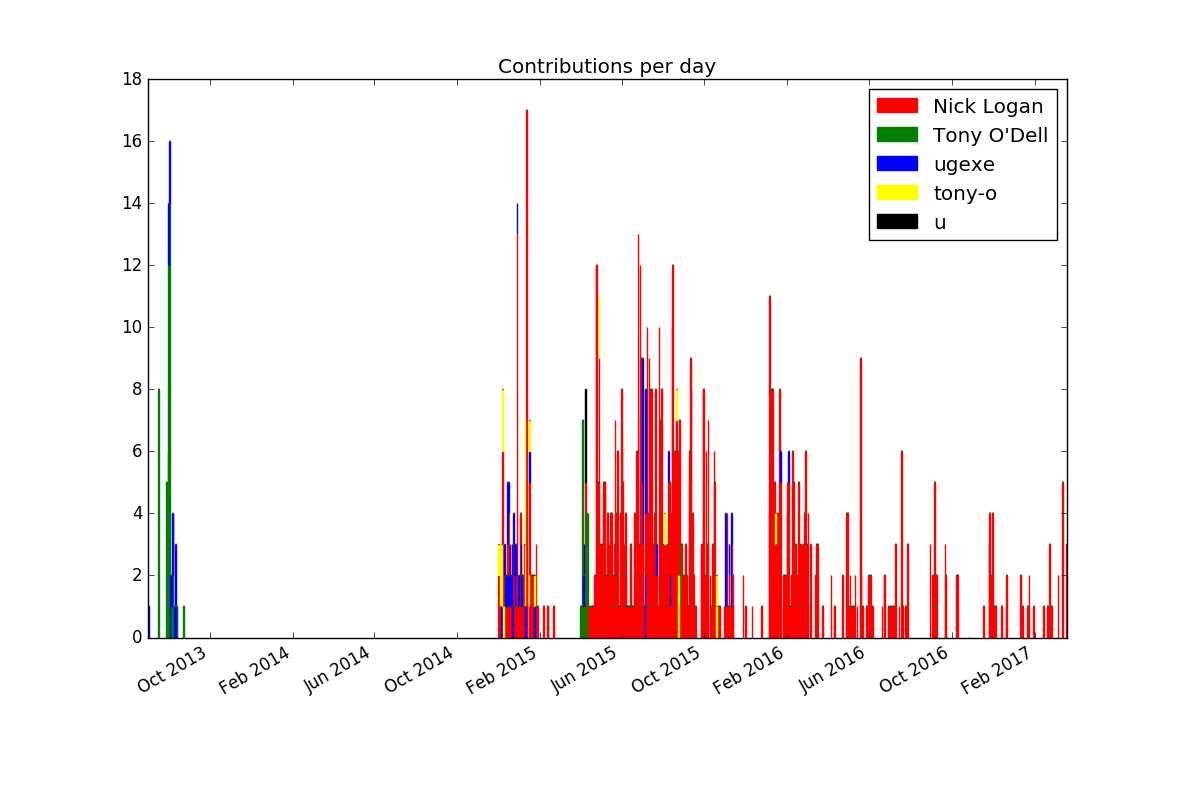# Perl 6 By Example: Stacked Plots with MatplotlibThis blog post is part of my ongoing project to write a book about Perl 6.

If you're interested, either in this book project or any other Perl 6 book news, please sign up for the mailing list at the bottom of the article, or here. It will be low volume (less than an email per month, on average).

In a previous episode, we've explored plotting git statistics in Perl 6 using matplotlib.

Since I wasn't quite happy with the result, I want to explore using stacked plots for presenting the same information. In a regular plot, the y coordiante of each plotted value is proportional to its value. In a stacked plot, it is the distance to the previous value that is proportional to its value. This is nice for values that add up to a total that is also interesting.

Matplotlib offers a method called `stackplot` for that. Contrary to multiple `plot` calls on subplot object, it requires a shared x axis for all data series. So we must construct one array for each author of git commits, where dates with no value come out as zero.

As a reminder, this is what the logic for extracting the stats looked like in the first place:

``````my \$proc = run :out, <git log --date=short --pretty=format:%ad!%an>;
my (%total, %by-author, %dates);
for \$proc.out.lines -> \$line {
my ( \$date, \$author ) = \$line.split: '!', 2;
%total{\$author}++;
%by-author{\$author}{\$date}++;
%dates{\$date}++;
}
``````

And some infrastructure for plotting with matplotlib:

``````my \$py = Inline::Python.new;
\$py.run('import datetime');
\$py.run('import matplotlib.pyplot');
sub plot(Str \$name, |c) {
\$py.call('matplotlib.pyplot', \$name, |c);
}
sub pydate(Str \$d) {
\$py.call('datetime', 'date', \$d.split('-').map(*.Int));
}

my (\$figure, \$subplots) = plot('subplots');
\$figure.autofmt_xdate();
``````

So now we have to construct an array of arrays, where each inner array has the values for one author:

``````my @dates = %dates.keys.sort;
my @stack = \$[] xx @top-authors;

for @dates -> \$d {
for @top-authors.kv -> \$idx, \$author {
@stack[\$idx].push: %by-author{\$author}{\$d} // 0;
}
}
``````

Now plotting becomes a simple matter of a method call, followed by the usual commands adding a title and showing the plot:

``````\$subplots.stackplot(\$[@dates.map(&pydate)], @stack);
plot('title', 'Contributions per day');
plot('show');
``````

The result (again run on the zef source repository) is this:Comparing this to the previous visualization reveals a discrepancy: There were no commits in 2014, and yet the stacked plot makes it appear this way. In fact, the previous plots would have shown the same "alternative facts" if we had chosen lines instead of points. It comes from matplotlib (like nearly all plotting libraries) interpolates linearly between data points. But in our case, a date with no data points means zero commits happened on that date.

To communicate this to matplotlib, we must explicitly insert zero values for missing dates. This can be achieved by replacing

``````my @dates = %dates.keys.sort;
``````

with the line

``````my @dates = %dates.keys.minmax;
``````

The `minmax` method finds the minimal and maximal values, and returns them in a Range. Assigning the range to an array turns it into an array of all values between the minimal and the maximal value. The logic for assembling the `@stack` variable already maps missing values to zero.

The result looks a bit better, but still far from perfect:Thinking more about the problem, contributions from separate days should not be joined together, because it produces misleading results. Matplotlib doesn't support adding a legend automatically to stacked plots, so this seems to be to be a dead end.

Since a dot plot didn't work very well, let's try a different kind of plot that represents each data point separately: a bar chart, or more specifically, a stacked bar chart. Matplotlib offers the `bar` plotting method, and a named parameter `bottom` can be used to generate the stacking:

``````my @dates = %dates.keys.sort;
my @stack = \$[] xx @top-authors;
my @bottom = \$[] xx @top-authors;

for @dates -> \$d {
my \$bottom = 0;
for @top-authors.kv -> \$idx, \$author {
@bottom[\$idx].push: \$bottom;
my \$value = %by-author{\$author}{\$d} // 0;
@stack[\$idx].push: \$value;
\$bottom += \$value;
}
}
``````

We need to supply color names ourselves, and set the edge color of the bars to the same color, otherwise the black edge color dominates the result:

``````my \$width = 1.0;
my @colors = <red green blue yellow black>;
my @plots;

for @top-authors.kv -> \$idx, \$author {
@plots.push: plot(
'bar',
\$[@dates.map(&pydate)],
@stack[\$idx],
\$width,
bottom => @bottom[\$idx],
color => @colors[\$idx],
edgecolor => @colors[\$idx],
);
}
plot('legend', \$@plots, \$@top-authors);

plot('title', 'Contributions per day');
plot('show');
``````

This produces the first plot that's actually informative and not misleading (provided you're not color blind):If you want to improve the result further, you could experiment with limiting the number of bars by lumping together contributions by week or month (or maybe `\$n`-day period).

Next, we'll investigate ways to make the matplotlib API more idiomatic to use from Perl 6 code.

## Subscribe to the Perl 6 book mailing list

* indicates required